# Linear Regression Outline Linear Regression Analysis Linear trend

• Slides: 14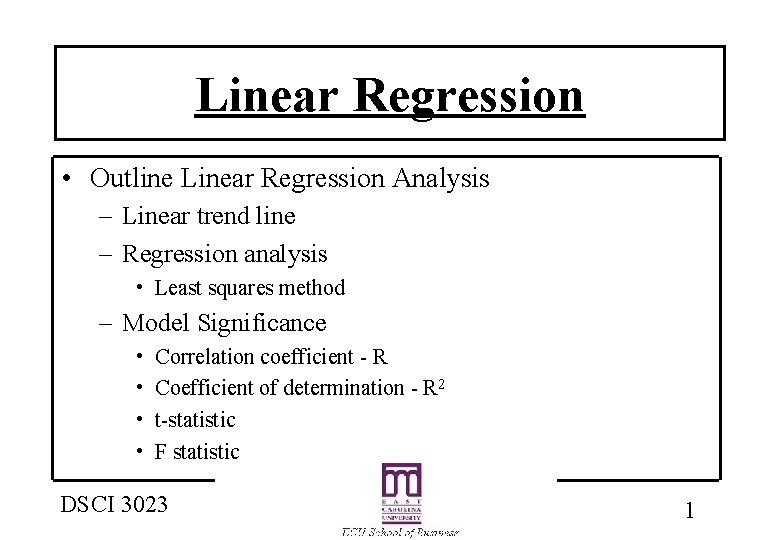Linear Regression • Outline Linear Regression Analysis – Linear trend line – Regression analysis • Least squares method – Model Significance • • Correlation coefficient - R Coefficient of determination - R 2 t-statistic F statistic DSCI 3023 1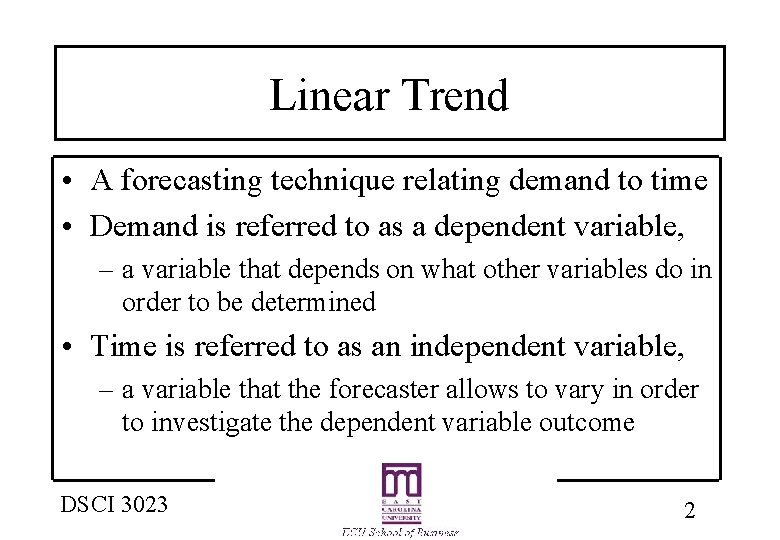Linear Trend • A forecasting technique relating demand to time • Demand is referred to as a dependent variable, – a variable that depends on what other variables do in order to be determined • Time is referred to as an independent variable, – a variable that the forecaster allows to vary in order to investigate the dependent variable outcome DSCI 3023 2Linear Trend • Linear regression takes on the form y = a + bx y = demand x = time • A forecaster allows time to vary and investigates the demands that the equation produces • A regression line can be calculated using what is called the least squares method DSCI 3023 3Why Linear Trend? • Why do forecasters chose a linear relationship? – Simple representation – Ease of use – Ease of calculations – Many relationships in the real world are linear – Start simple and eliminate relationships which do not work DSCI 3023 4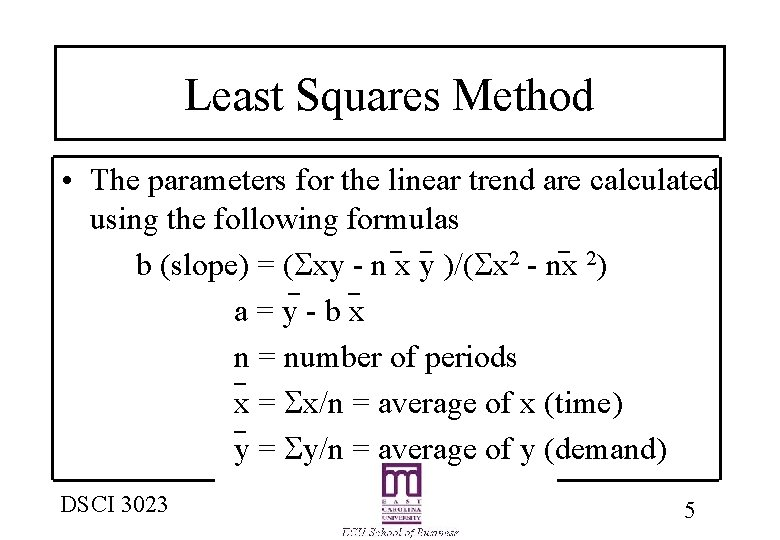Least Squares Method • The parameters for the linear trend are calculated using the following formulas b (slope) = ( xy - n x y )/( x 2 - nx 2) a=y-bx n = number of periods x = x/n = average of x (time) y = y/n = average of y (demand) DSCI 3023 5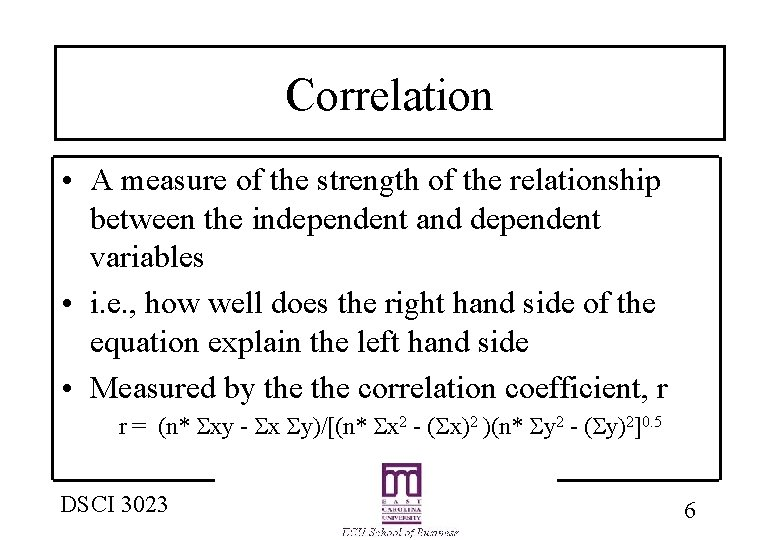Correlation • A measure of the strength of the relationship between the independent and dependent variables • i. e. , how well does the right hand side of the equation explain the left hand side • Measured by the correlation coefficient, r r = (n* xy - x y)/[(n* x 2 - ( x)2 )(n* y 2 - ( y)2]0. 5 DSCI 3023 6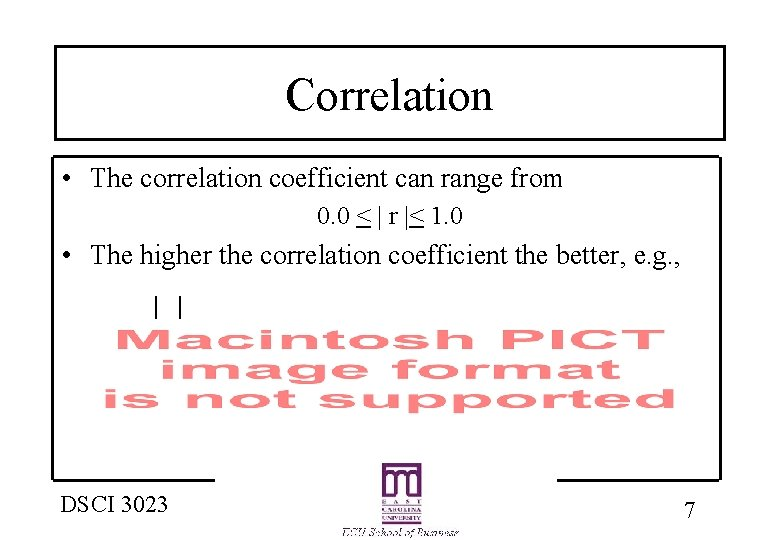Correlation • The correlation coefficient can range from 0. 0 < | r |< 1. 0 • The higher the correlation coefficient the better, e. g. , DSCI 3023 7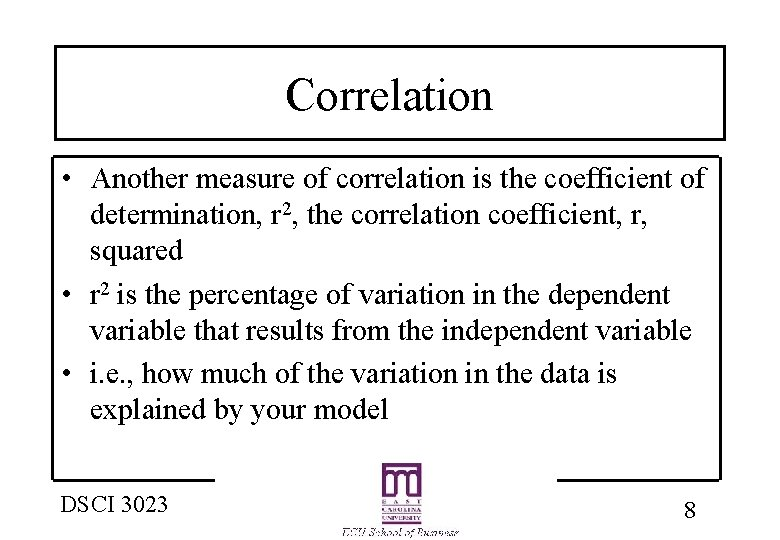Correlation • Another measure of correlation is the coefficient of determination, r 2, the correlation coefficient, r, squared • r 2 is the percentage of variation in the dependent variable that results from the independent variable • i. e. , how much of the variation in the data is explained by your model DSCI 3023 8Multiple Regression • A more powerful extension of linear regression • Multiple regression relates a dependent variable to more than one independent variables • e. g. , new housing may be a function of several independent variables – – interest rate population housing prices income DSCI 3023 9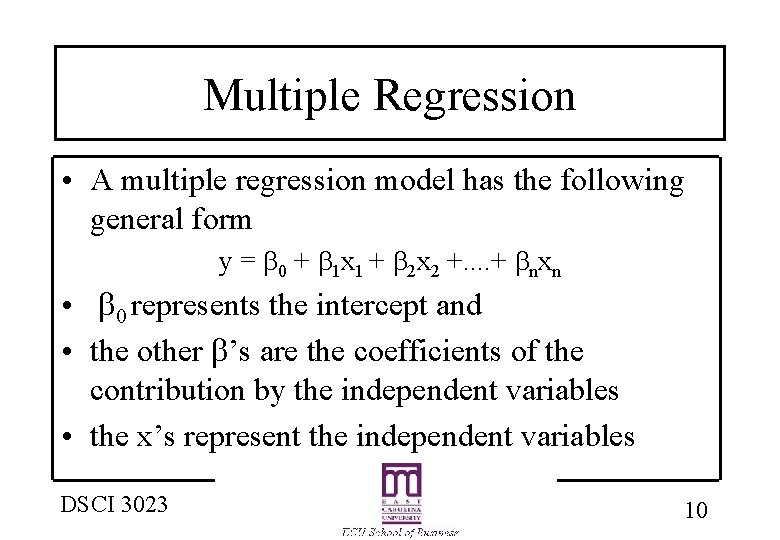Multiple Regression • A multiple regression model has the following general form y = 0 + 1 x 1 + 2 x 2 +. . + nxn • 0 represents the intercept and • the other ’s are the coefficients of the contribution by the independent variables • the x’s represent the independent variables DSCI 3023 10Multiple Regression Performance • How a multiple regression model performs is measured the same way as a linear regression • r 2 is calculated and interpreted • A t-statistic is also calculated for each to measure each independent variables significance • The t-stat is calculated as follows t-stat = i/sse i DSCI 3023 11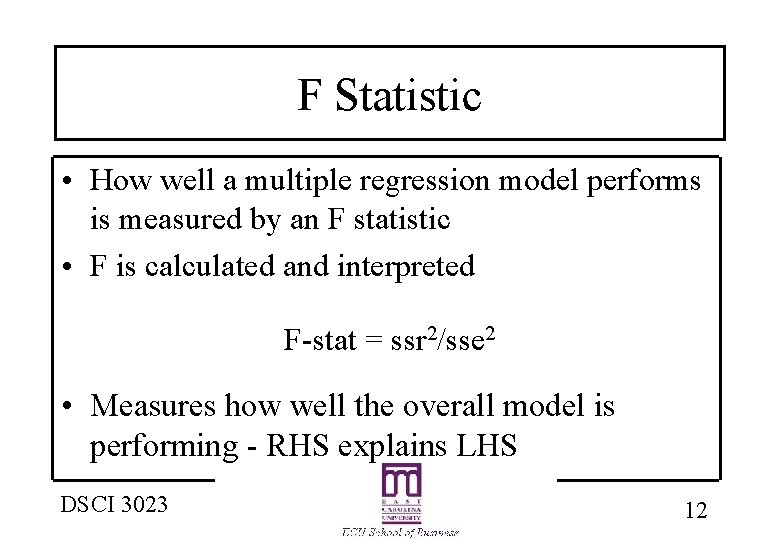F Statistic • How well a multiple regression model performs is measured by an F statistic • F is calculated and interpreted F-stat = ssr 2/sse 2 • Measures how well the overall model is performing - RHS explains LHS DSCI 3023 12Least Squares Example • Calculate the mean of x and the mean of y • Calculate the slope using the LS formula • Calculate the intercept using the LS formula • Plot the LS line and the data • Interpret the relationship to the data DSCI 3023 13Comparison of LS and Time Series • Use the same example for lumber sales • Forecast lumber sales using the linear regression model developed and the building permit data supplied • Also forecast using a 3 -MA • Calculate MAD for each method and compare DSCI 3023 14